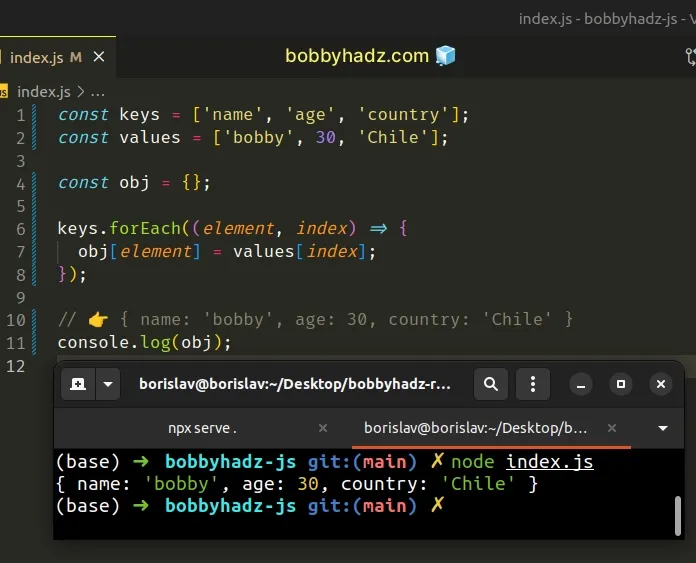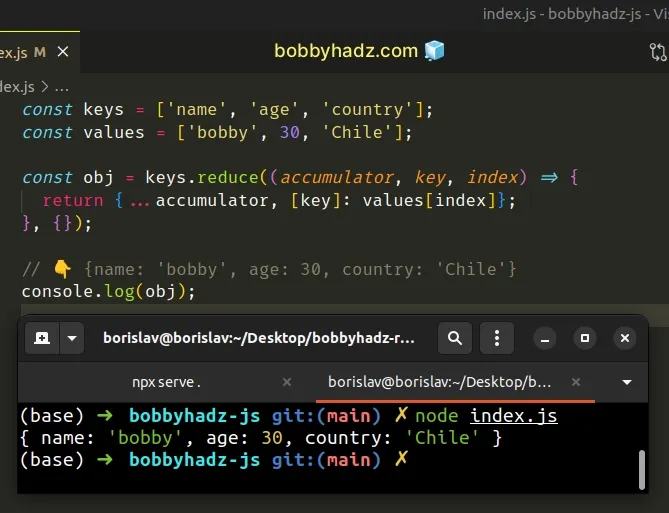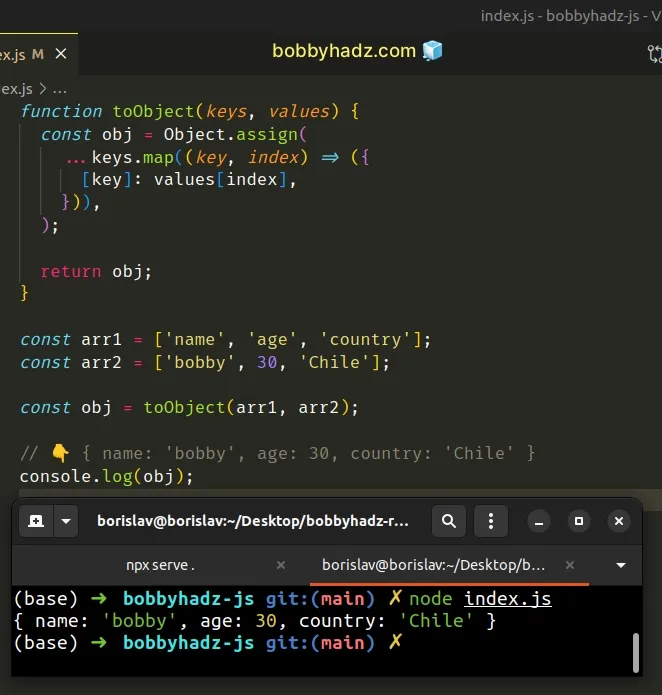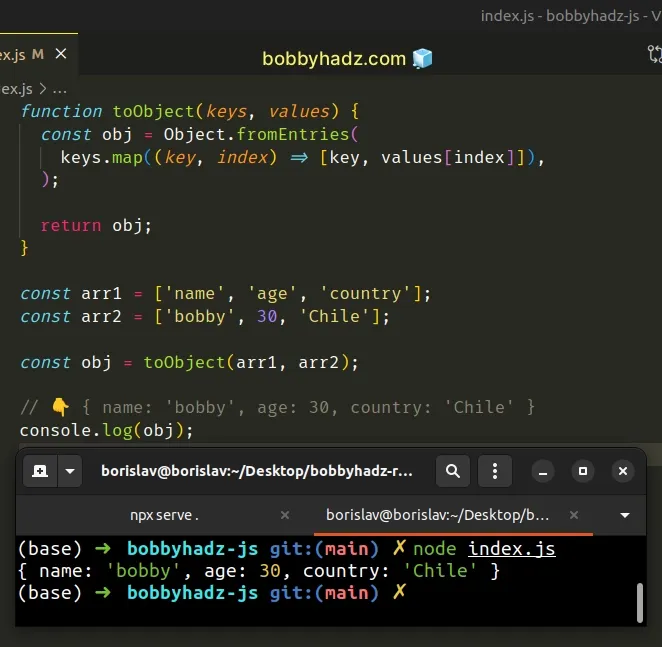# Create an Object from Two Arrays in JavaScriptLast updated: Jan 7, 2023
4 min## #Create an Object from Two Arrays in JavaScript

To create an object from two arrays:

1. Use the `Array.forEach()` method to iterate over the array of keys.
2. Use the index to access the element from the values array.
3. On each iteration, assign the key-value pair to an object.
index.js
```Copied!```const keys = ['name', 'age', 'country'];
const values = ['bobby', 30, 'Chile'];

const obj = {};

keys.forEach((element, index) => {
obj[element] = values[index];
});

// 👉️ { name: 'bobby', age: 30, country: 'Chile' }
console.log(obj);
``````The function we passed to the Array.forEach method gets called with each element in the array.

The function gets passed the following 3 parameters:

1. The current `element`.
2. The `index` of the iteration.
3. The `array` itself.
We made use of the index to access the value in the second array and assigned the key-value pair to an object.

After the method has finished iterating, the object contains the key-value pairs from the two arrays.

If you have to do this often, define a reusable function.

index.js
```Copied!```function toObject(keys, values) {
const obj = {};

keys.forEach((element, index) => {
obj[element] = values[index];
});

return obj;
}

const arr1 = ['name', 'age', 'country'];
const arr2 = ['bobby', 30, 'Chile'];

const obj = toObject(arr1, arr2);

// 👇️ { name: 'bobby', age: 30, country: 'Chile' }
console.log(obj);
``````

The `toObject()` function takes two arrays as parameters, creates an object from the two arrays and returns the result.

An alternative, but more functional approach is to use the Array.reduce method.

## #Create an Object from Two Arrays using Array.reduce()

This is a three-step process:

1. Use the `reduce()` method to iterate over the keys array.
2. Provide an empty object as the initial value for the accumulator.
3. Using the index, assign the key-value pair to the accumulated object.
index.js
```Copied!```const keys = ['name', 'age', 'country'];
const values = ['bobby', 30, 'Chile'];

const obj = keys.reduce((accumulator, key, index) => {
return {...accumulator, [key]: values[index]};
}, {});

// 👇️️ {name: 'bobby', age: 30, country: 'Chile'}
console.log(obj);
``````The function we passed to the `reduce()` method gets called for each element in the array.

We provided an empty object as the initial value of the `accumulator` variable.

On each iteration, we use the spread syntax (...) to unpack the key-value pairs of the `accumulator` into a new object, then add the current key-value pair and return the result.

Once the `reduce()` method has iterated over the entire array, the `accumulator` object contains the key-value pairs from the two arrays.

If you have to do this often, define a reusable function.

index.js
```Copied!```function toObject(keys, values) {
const obj = keys.reduce((accumulator, key, index) => {
return {...accumulator, [key]: values[index]};
}, {});

return obj;
}

const arr1 = ['name', 'age', 'country'];
const arr2 = ['bobby', 30, 'Chile'];

const obj = toObject(arr1, arr2);

// 👇️ { name: 'bobby', age: 30, country: 'Chile' }
console.log(obj);
``````

The function takes 2 arrays as parameters and creates an object from the two arrays.

## #Create an Object from Two Arrays using Object.assign()

This is a three-step process:

1. Use the `Array.map()` method to iterate over the array of keys.
2. Return an object containing the current key-value pair on each iteration.
3. Use the `Object.assign()` method to merge the objects.
index.js
```Copied!```function toObject(keys, values) {
const obj = Object.assign(
...keys.map((key, index) => ({
[key]: values[index],
})),
);

return obj;
}

const arr1 = ['name', 'age', 'country'];
const arr2 = ['bobby', 30, 'Chile'];

const obj = toObject(arr1, arr2);

// 👇️ { name: 'bobby', age: 30, country: 'Chile' }
console.log(obj);
``````The function we passed to the Array.map() method gets called with each element in the array.

On each iteration, we return an object containing the key from the first array and the value from the second array.

index.js
```Copied!```const arr1 = ['name', 'age', 'country'];
const arr2 = ['bobby', 30, 'Chile'];

// 👇️ { name: 'bobby' } { age: 30 } { country: 'Chile' }
console.log(
...arr1.map((key, index) => ({
[key]: arr2[index],
})),
);
``````

The `map()` method returns a new array containing the values returned from the callback function.

The last step is to use the `Object.assign()` method to merge the objects into a single object.

## #Create an Object from Two Arrays using Object.fromEntries()

This is a three-step process:

1. Use the `Array.map()` method to iterate over the keys array.
2. Return an array containing the current key-value pair on each iteration.
3. Use the `Object.fromEntries()` method to convert the array to an object.
index.js
```Copied!```function toObject(keys, values) {
const obj = Object.fromEntries(
keys.map((key, index) => [key, values[index]]),
);

return obj;
}

const arr1 = ['name', 'age', 'country'];
const arr2 = ['bobby', 30, 'Chile'];

const obj = toObject(arr1, arr2);

// 👇️ { name: 'bobby', age: 30, country: 'Chile' }
console.log(obj);
``````We used the `Array.map()` method to create an array of key-value pair arrays.

index.js
```Copied!```const arr1 = ['name', 'age', 'country'];
const arr2 = ['bobby', 30, 'Chile'];

// 👇️ [ [ 'name', 'bobby' ], [ 'age', 30 ], [ 'country', 'Chile' ] ]
console.log(arr1.map((key, index) => [key, arr2[index]]));
``````

The last step is to pass the array of key-value pairs to the `Object.fromEntries()` method.

The Object.fromEntries transforms a list of key-value pairs into an object.

This only works if you have a two-dimensional array where each subarray contains 2 elements - the key and the value.